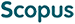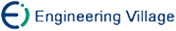Dong Rongsheng, Zhang Xinkai, Liu Huadong, Gu Tianlong. Representation and Operations Research of k\+2-MDD in Large-Scale Graph Data[J]. Journal of Computer Research and Development, 2016, 53(12): 2783-2792. DOI: 10.7544/issn1000-1239.2016.20160589
 Citation: Dong Rongsheng, Zhang Xinkai, Liu Huadong, Gu Tianlong. Representation and Operations Research of k\+2-MDD in Large-Scale Graph Data[J]. Journal of Computer Research and Development, 2016, 53(12): 2783-2792. DOI: 10.7544/issn1000-1239.2016.20160589# Representation and Operations Research of k\+2-MDD in Large-Scale Graph Data• Efficient and compact representation and operation of graph data which contains hundreds of millions of vertices and edges are the basis of analyzing and processing the large scale of graph data. Aiming at the problem, this paper proposes a representation of large-scale graph data based on the decision diagram, that is k\+2-MDD, providing the initialization of k\+2-MDD and the basic operation such as the edge query, inner(outer) neighbor query, finding out(in)-degree, adding(deleting) edge, etc. The representation method is optimized and improved on the basis of k\+2 tree, and after dividing the adjacency matrix of graph into k\+2, it is stored with the multi valued decision diagram, so as to achieve a more compact storage structure. According to the experimental results of a series of real Web graph and the social network graph data (cnr-2000, dewiki-2013, etc.) derived from the LAW laboratory at the University of Milan, it can be seen that the number of k\+2-MDD’ nodes is only 259%-451% of the k\+2 tree, which achieving the desired effect. According to the experimental results of random graphs, it can be seen that the k\+2-MDD structure is not only suitable for sparse graphs, but also for dense graphs. The graph data of k\+2-MDD shows that both containing the compact and query efficiency representation of k\+2 tree and realizing the efficient operation of the graph model can thus achieve the unity of description and computing power.
•Turn off MathJax
Article Contents/DownLoad:  Full-Size Img  PowerPoint